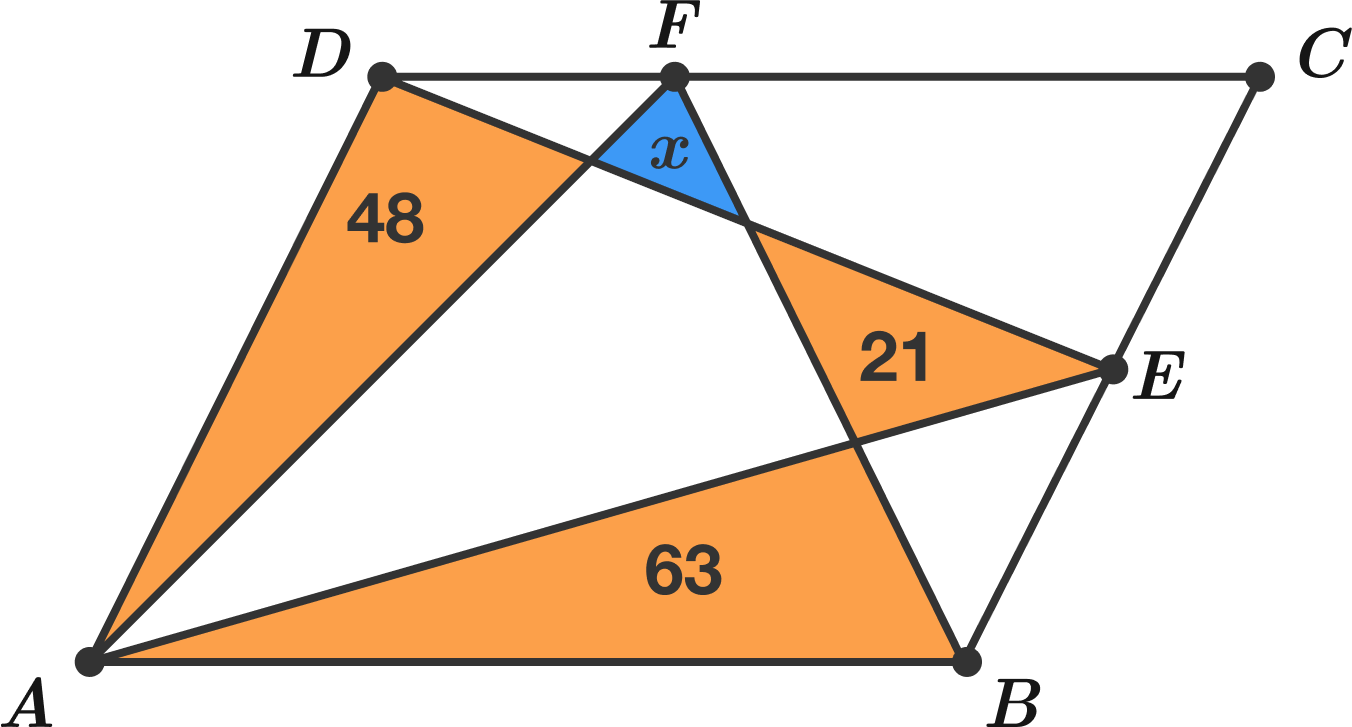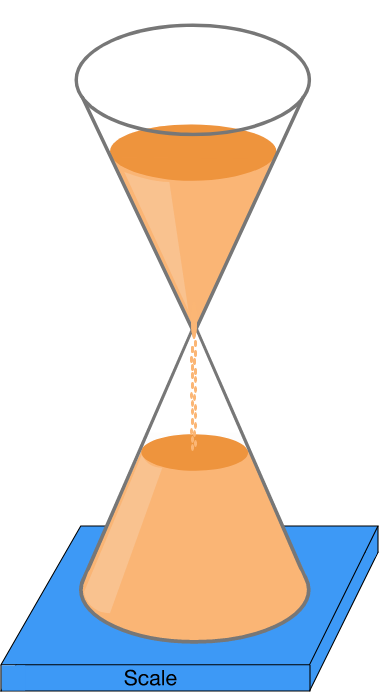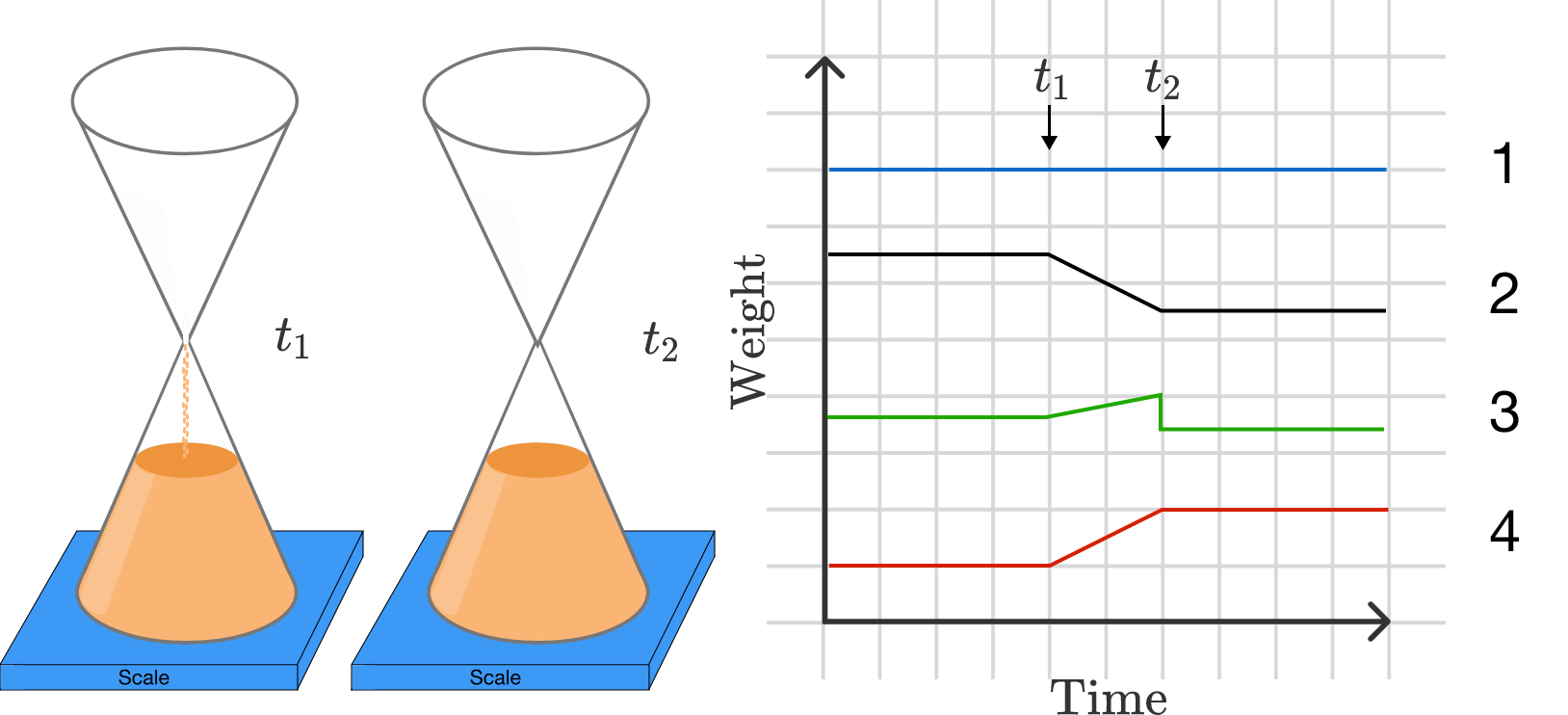# Problems of the Week

Contribute a problem

# 2018-05-21 Intermediate

The line segments shown divide parallelogram $ABCD$ into 8 regions, and the areas of the orange triangles are 48, 21, and 63.

What is the area of the blue triangle?The weight of this hourglass is monitored constantly.The last grain of sand falls from the top of the hourglass at $t_1$, and hits the bottom at $t_2$.

Which line best represents the readout of the weight on the scale?Is there a positive integer divisible by 2018 which only consists of the digits 3 and 0 (in base 10)?

If one of the two series below is equal to 2, what is the value of the other one?

$\begin{cases} 1 + x + x^2 + x^3 + \cdots \\\\ x + 2x^2 + 3x^3 + 4x^4 + \cdots \end{cases}$

Your friend, David Hilbert, runs a casino with space for an infinite number of guests. He installs a slot machine with the following properties:

• Each guest at the casino plays one round at the slot machine after all the guests have arrived.
• If there are $n$ guests at the casino, then the odds of winning the slot machine are always $\frac{1}{n}$ for all of them.
• When a guest wins, they get all of the money in the machine.

To understand how profitable this machine is, he wants to know the probability that no one wins the slot machine.

If David fills up his casino with a number of guests approaching infinity, what does this probability approach?

×